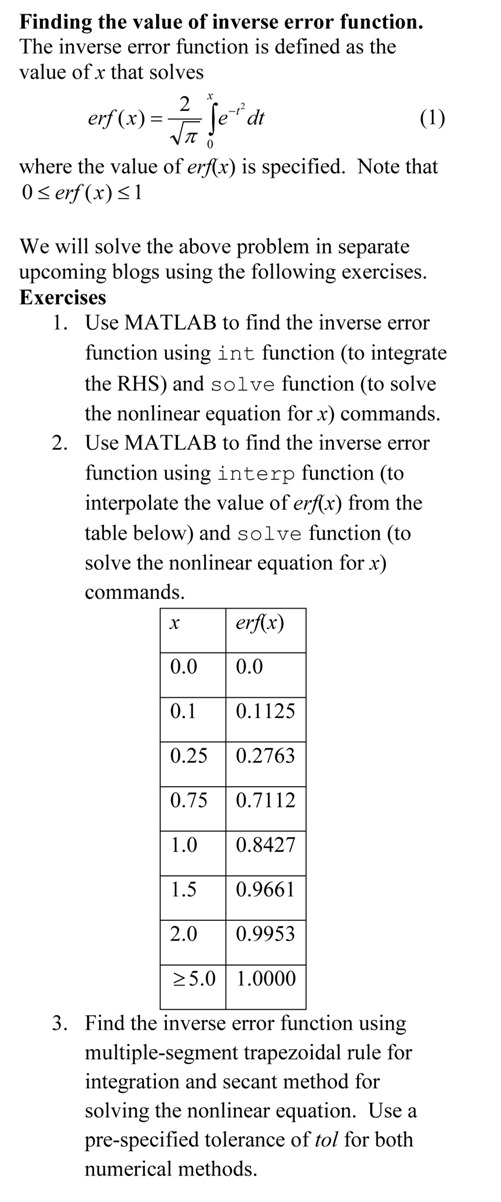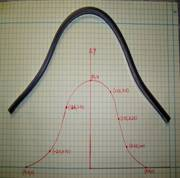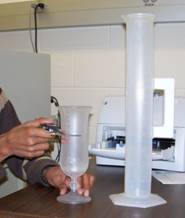## Do we have to setup all 3n equations for the n quadratic splines for (n+1) data points?

QUESTION ASKED BY STUDENT: For linear splines, we still find each line individually. In quadratic, we are forced to find every equation at once, correct? Which is where the matrix comes from?

ANSWER: That sounds right, but one can find quadratic equations individually also .
How?
If the first spline is linear, then that spline can be found by the two points it goes through.
Then the second spline goes thru two points, and that gives two equations.  The slope of the second spline is same as the slope of first spline at the common interior point, and the first spline is known. This gives the third eqn.  This process can be repeated for each following spline.

____________________________________

This post is brought to you by Holistic Numerical Methods: Numerical Methods for the STEM undergraduate at http://nm.mathforcollege.com, the textbook on Numerical Methods with Applications available from the lulu storefront, the textbook on Introduction to Programming Concepts Using MATLAB, and the YouTube video lectures available at http://nm.mathforcollege.com/videos.  Subscribe to the blog via a reader or email to stay updated with this blog. Let the information follow you.

## Inverse error function using interpolation

In the previous post, http://autarkaw.wordpress.com/2010/09/01/using-int-and-solve-to-find-inverse-error-function-in-matlab/, we found the inverse error function by using the integral and solve MATLAB functions.  In this blog, we find the inverse error function by using interpolation.

The value of erf(x) is given at discrete data points of x, and we use spline interpolation to find the value of x at a given value of erf(x).  The given data points of (x,erf(x)) are (0,0), (0.1,0.1125), (0.25,0.2763), (0.75,0.7112), (1.0,0.8427), (1.5,0.9661), (2.0,0.9953), (5.0,1.000).

It is better to download (right click and save target) the program as single quotes in the pasted version do not translate properly when pasted into a mfile editor of MATLAB or you can read the html version for clarity and sample output.

%% FINDING INVERSE ERROR FUNCTION
% In a previous blog at autarkaw.wordpress.com (Sep 1, 2010), we set up a
% nonlinear equation to find the inverse error function.
% In this blog, we will solve this equation
% by using interpolation.
% The problem is given at
% http://nm.mathforcollege.com/blog/inverseerror.pdf
% and we are solving Exercise 2 of the pdf file.

%% TOPIC
% Finding inverse error function

%% SUMMARY

% Language : Matlab 2008a;
% Authors : Autar Kaw;
% Mfile available at
% http://nm.mathforcollege.com/blog/inverse_erf_interp_matlab.m;
% Last Revised : October 4 2010
% Abstract: This program shows you how to find the inverse error function
% using interpolation
clc
clear all

%% INTRODUCTION

disp(‘ABSTRACT’)
disp(‘   This program shows you how to’)
disp(‘   find the inverse error function’)
disp(‘ ‘)
disp(‘AUTHOR’)
disp(‘   Autar K Kaw of http://autarkaw.wordpress.com’)
disp(‘ ‘)
disp(‘MFILE SOURCE’)
disp(‘ http://nm.mathforcollege.com/blog/inverse_erf_interp_matlab.m’)
disp(‘  ‘)
disp(‘PROBLEM STATEMENT’)
disp(‘ http://nm.mathforcollege.com/blog/inverseerror.pdf  Exercise 2′)
disp(‘ ‘)
disp(‘LAST REVISED’)
disp(‘   October 4, 2010’)
disp(‘ ‘)

%% INPUTS
% Value of error function
erfx=0.1125;
% Table of erf(x) vs x
xx=[0  0.1  0.25  0.75  1.0  1.5  2.0  5.0];
erfxx=[0  0.1125  0.2763  0.7112  0.8427  0.9661  0.9953  1.0000];

%% DISPLAYING INPUTS

disp(‘INPUTS’)
fprintf(‘ Inverse error function is to be found for= %g’,erfx)
disp(‘  ‘)
disp(‘ Given erf(x) vs x values’)
disp(‘_______________________’)
disp(‘    x          erfx  ‘)
disp(‘________________________’)
dataval=[xx;erfxx]’;
disp(dataval)

%% CODE
if erfx>1.0 | erfx<0
disp(‘Invalid value. erf(x) only takes values in [0,1] range’)
else
inverse_erf=interp1(erfxx,xx,erfx,’cubic’);
%% DISPLAYING OUTPUTS

disp(‘OUTPUTS’)
fprintf(‘ Value of inverse error func from this mfile is= %g’,inverse_erf)
fprintf(‘ \n Value of inverse error func from MATLAB is    = %g’,erfinv(erfx))
disp(‘  ‘)
end

__________________________________________________

This post is brought to you by

Holistic Numerical Methods: Numerical Methods for the STEM undergraduate at http://nm.mathforcollege.com,
the textbook on Numerical Methods with Applications available from the lulu storefront,
the textbook on Introduction to Programming Concepts Using MATLAB, and
the YouTube video lectures available at http://nm.mathforcollege.com/videos

## Finding the inverse error functionThis post is brought to you by Holistic Numerical Methods: Numerical Methods for the STEM undergraduate at http://nm.mathforcollege.com, the textbook on Numerical Methods with Applications available from the lulu storefront, and the YouTube video lectures available at http://nm.mathforcollege.com/videos and http://www.youtube.com/numericalmethodsguy

## How do I do spline interpolation in MATLAB?

Many students ask me how do I do this or that in MATLAB.  So I thought why not have a small series of my next few blogs do that.  In this blog, I show you how to conduct spline interpolation.

• The MATLAB program link is here.
• The HTML version of the MATLAB program is here.
• DO NOT COPY AND PASTE THE PROGRAM BELOW BECAUSE THE SINGLE QUOTES DO NOT TRANSLATE TO THE CORRECT SINGLE QUOTES IN MATLAB EDITOR.  DOWNLOAD THE MATLAB PROGRAM INSTEAD

%% HOW DO I DO THAT IN MATLAB SERIES?
% In this series, I am answering questions that students have asked
% me about MATLAB.  Most of the questions relate to a mathematical
% procedure.

%% TOPIC
% How do I do spline interpolation?

%% SUMMARY

% Language : Matlab 2008a;
% Authors : Autar Kaw;
% Mfile available at
% http://nm.mathforcollege.com/blog/interpolate_spline.m;
% Last Revised : June 20, 2009;
% Abstract: This program shows you how to do spline interpolation?
%           .
clc
clear all
clf

%% INTRODUCTION

disp(‘ABSTRACT’)
disp(‘   This program shows you how to do spline interpolation?’)
disp(‘ ‘)
disp(‘AUTHOR’)
disp(‘   Autar K Kaw of http://autarkaw.wordpress.com’)
disp(‘ ‘)
disp(‘MFILE SOURCE’)
disp(‘   http://nm.mathforcollege.com/blog/interpolation_spline.m’)
disp(‘ ‘)
disp(‘LAST REVISED’)
disp(‘   June 20, 2009’)
disp(‘ ‘)

%% INPUTS
% y vs x data to interpolate
% x data
x=[-1  -0.75  -0.5  -0.25   0.25  0.50 0.75  1];
% ydata
y=[-0.5  -0.5  -0.5  -0.5   0.5  0.5  0.5  0.5];
% Where do you want to interpolate at
xin=[-0.8  -0.7  0.7  0.8];
%% DISPLAYING INPUTS
disp(‘INPUTS’)
disp(‘The x data’)
x
disp(‘The y data’)
y
disp(‘The x values where you want to find the interpolated values’)
xin
disp(‘  ‘)

%% THE CODE
% Fitting to spline – it is cubic splines
yin=spline(x,y,xin);
% This is only for plotting the spline interpolants
% Find the number of data points
n=length(x);
xplot=x(1):(x(n)-x(1))/10000:x(n);
yplot=spline(x,y,xplot);

%% DISPLAYING OUTPUTS
disp(‘  ‘)
disp(‘OUTPUTS’)
disp(‘x values at which function is to be interpolated’)
xin
disp(‘y values at the xin values’)
yin
xlabel(‘x’);
ylabel(‘y’);
title(‘y vs x ‘);
plot(x,y,’o’,’MarkerSize’,10,’MarkerEdgeColor’,’b’,’MarkerFaceColor’,’b’)
hold on
plot(xin,yin,’o’,’MarkerSize’,10,’MarkerEdgeColor’,’r’,’MarkerFaceColor’,’r’)
hold on
plot(xplot,yplot,’LineWidth’,2)
legend(‘Points given’,’Points found’,’Spline Curve’,’Location’,’East’)
hold off
disp(‘  ‘)

This post is brought to you by Holistic Numerical Methods: Numerical Methods for the STEM undergraduate at http://nm.mathforcollege.com, the textbook on Numerical Methods with Applications available from the lulu storefront, and the YouTube video lectures available at http://nm.mathforcollege.com/videos

## How do I do polynomial interpolation in MATLAB

Many students ask me how do I do this or that in MATLAB.  So I thought why not have a small series of my next few blogs do that.  In this blog, I show you how to do polynomial interpolation.

• The MATLAB program link is here.
• The HTML version of the MATLAB program is here.
• DO NOT COPY AND PASTE THE PROGRAM BELOW BECAUSE THE SINGLE QUOTES DO NOT TRANSLATE TO THE CORRECT SINGLE QUOTES IN MATLAB EDITOR.  DOWNLOAD THE MATLAB PROGRAM INSTEAD

%% HOW DO I DO THAT IN MATLAB SERIES?
% In this series, I am answering questions that students have asked
% me about MATLAB.  Most of the questions relate to a mathematical
% procedure.

%% TOPIC
% How do I do polynomial interpolation?

%% SUMMARY

% Language : Matlab 2008a;
% Authors : Autar Kaw;
% Mfile available at
% http://nm.mathforcollege.com/blog/interpolate_polynomial.m;
% Last Revised : June 10, 2009;
% Abstract: This program shows you how to do polynomial interpolation?
%           .
clc
clear all
clf

%% INTRODUCTION

disp(‘ABSTRACT’)
disp(‘   This program shows you how to do polynomial interpolation?’)
disp(‘ ‘)
disp(‘AUTHOR’)
disp(‘   Autar K Kaw of http://autarkaw.wordpress.com’)
disp(‘ ‘)
disp(‘MFILE SOURCE’)
disp(‘   http://nm.mathforcollege.com/blog/interpolation_polynomial.m’)
disp(‘ ‘)
disp(‘LAST REVISED’)
disp(‘   June 10, 2009’)
disp(‘ ‘)

%% INPUTS
% y vs x data to interpolate
% x data
x=[-1  -0.6  -0.2  0.2  0.6  1];
% ydata
y=[0.0385    0.1000    0.5000    0.5000    0.1000    0.0385];
% Where do you want to interpolate at
xin=[-0.8  -0.7  0.7  0.8];
%% DISPLAYING INPUTS
disp(‘INPUTS’)
disp(‘The x data’)
x
disp(‘The y data’)
y
disp(‘The x values where you want to find the interpolated values’)
xin
disp(‘  ‘)

%% THE CODE
% Find the number of data points
n=length(x);
% Fitting to polynomial of order m=n-1
m=n-1
% pp consists of the coefficients of the polynomial
% pp(1)*x^m+pp(2)*x^m+…….+pp(m)
% pp(1) is coefficient of x^m
% pp(2) is coefficient of x^(m-1)
% and so on
pp=polyfit(x,y,m);
% Getting the values at xin
yin=polyval(pp,xin);
% This is only for plotting the interpolating polynomial
xplot=x(1):(x(n)-x(1))/10000:x(n);
yplot=polyval(pp,xplot);

%% DISPLAYING OUTPUTS
disp(‘  ‘)
disp(‘OUTPUTS’)
disp(‘x values at which function is to be interpolated’)
xin
disp(‘y values at the xin values’)
yin
disp(‘These are the coefficients of the polynomial interpolant’)
disp(‘pp(1) is coefficient of x^m, pp(2) is coefficient of x^(m-1) and so on’)
fprintf(‘Order of polynomial m =%g’,m)
pp
xlabel(‘x’);
ylabel(‘y’);
title(‘y vs x ‘);
plot(x,y,’o’,’MarkerSize’,10,’MarkerEdgeColor’,’b’,’MarkerFaceColor’,’b’)
hold on
plot(xin,yin,’o’,’MarkerSize’,10,’MarkerEdgeColor’,’k’,’MarkerFaceColor’,’k’)
hold on
plot(xplot,yplot,’LineWidth’,2)
legend(‘Points given’,’Points found’,’Polynomial Curve’)
hold off
disp(‘  ‘)

This post is brought to you by Holistic Numerical Methods: Numerical Methods for the STEM undergraduate at http://nm.mathforcollege.com, the textbook on Numerical Methods with Applications available from the lulu storefront, and the YouTube video lectures available at http://nm.mathforcollege.com/videos

## Experimental data for the length of curve experiment

In a previous post (click on the link on the left to learn fully about the experiment, and the assigned problems), I talkedabout an experiment we conduct in class to compare spline and polynomial interpolation.  If you do not want to conduct the experiment itself but want the (x,y) data to see for yourself how polynomial and spline interpolation compare, the data is given below.

Length of graduated flexible curve = 12″

The points on the x-y graph are as follows

(-4.1,0), (-2.6,1), (-2.0,2,2), (-1.6, 3.0), (-1,3.6), (0,3.9), (1.6,2.8), (3.2,0.4), (4.1,0)

________________________________________________________________________________________________

This post is brought to you by Holistic Numerical Methods: Numerical Methods for the STEM undergraduate at http://nm.mathforcollege.com.

An abridged (for low cost) book on Numerical Methods with Applications will be in print (includes problem sets, TOC, index) on December 10, 2008 and available at lulu storefront.

## Experiment for spline interpolation and integration

Background:

The motivation behind the experiment is to understand spline interpolation and numerical integration by finding the volume of water that can be held by a champagne glass.

What does the student do in the lab:

The student chooses one of the odd-shaped champagne glasses (Figure 1). The student measures the outer radius of the champagne glass at different known locations along the height. The student measures the thickness of the glass, so that he/she will be able to find the inner radius of the champagne glass at the locations he/she measured the outer radius. The student pours water to the brim in the champagne glass and checks how much volume the champagne glass holds.Exercises assigned to the students:
Use MATLAB to solve problems. Use comments, display commands and fprintf statements, sensible variable names and units to explain your work. Staple all the work in the following sequence. Use USCS system of units throughout.

1. Attach the data sheet on which you collected the data in class.
2. Find the spline interpolant that curve fits the radius vs height data.
3. Show the individual points and the spline interpolant of radius vs height on a single plot.
4. Find how much volume of water the champagne glass would hold.
5. Compare the above result from problem#4 to the actual volume.
6. In 100-200 words, type out your conclusions using a word processor. Any formulas should be shown using an equation editor. Any sketches need to be drawn using a drawing software such as Word Drawing. Any plots can be imported from MATLAB.

What materials do you need; where do I buy it; how much do the materials costs?

1. Champagne Glasses: These glasses, called the Hurricane Plastic Glasses, are available at www.poolsidepineapple.com, part nos. HUR-105, HUR-106, YAR-114. We used glasses made of plastic to avoid breakage. http:/www.poolsidepineapple.com/cart_pages/shopping%20page%20tropical.htm. You can try other places to buy the champagne glasses. About \$40 or so for about six pieces including S&H. Better yet, go to a cruise and get souvenir glasses. Whenever you do the experiment, you will remember the good times.
2. Graduated Cylinder: The graduated cylinder is available at http://scientificsonline.com/, part number 3036286. The cost of the cylinder is \$20+S&H.
3. Vernier Caliper: The caliper is available at http://mcmaster.com part number 20265A49. The cost of the vernier caliper is \$60+S&H.
4. Scale: Need to buy a thin scale for this. Any art-supplies store for \$2 or so.

_____________________________________________________

This post is brought to you by Holistic Numerical Methods: Numerical Methods for the STEM undergraduate at http://nm.mathforcollege.com

## Length of a curve experiment

In a previous post, I mentioned that I have incorporated experiments in my Numerical Methods course. Here I will discuss the second experiment.In this experiment, we find the length of two curves generated from the same points – one curve is a polynomial interpolant and another one is a spline interpolant.

Motivation behind the experiment: In 1901, Runge conducted a numerical experiment to show that higher order interpolation is a bad idea. It was shown that as you use higher order interpolants to approximate f(x)=1/(1+25x2) in [-1,1], the differences between the original function and the interpolants becomes worse. This concept also becomes the basis why we use splines rather than polynomial interpolation to find smooth paths to travel through several discrete points.

What do students do in the lab: A flexible curve (see Figure) of length 12″ made of lead-core construction with graduations in both millimeters and inches is provided. The student needs to draw a curve similar in shape to the Runge’s curve on the provided graphing paper as shown. It just needs to be similar in shape – the student can make the x-domain shorter and the maximum y-value larger or vice-versa. The student just needs to make sure that there is a one-to-one correspondence of values.

Assigned Exercises: Use MATLAB to solve problems (3 thru 6). Use comments, display commands and fprintf statements, sensible variable names and units to explain your work. Staple all the work in the following sequence.

1. Signed typed affidavit sheet.
2. Attach the plot you drew in the class. Choose several points (at least nine – do not need to be symmetric) along the curve, including the end points. Write out the co-ordinates on the graphing paper curve as shown in the figure.
3. Find the polynomial interpolant that curve fits the data. Output the coefficients of the polynomial.
4. Find the cubic spline interpolant that curve fits the data. Just show the work in the mfile.
5. Illustrate and show the individual points, polynomial and cubic spline interpolants on a single plot.
6. Find the length of the two interpolants – the polynomial and the spline interpolant. Calculate the relative difference between the length of each interpolant and the actual length of the flexible curve.
7. In 100-200 words, type out your conclusions using a word processor. Any formulas should be shown using an equation editor. Any sketches need to be drawn using a drawing software such as Word Drawing. Any plots can be imported from MATLAB.

Where to buy the items for the experiment:

1. Flexible curves – I bought these via internet at Art City. The brand name is Alvin Tru-Flex Graduated Flexible Curves. Prices range from \$5 to \$12. Shipping and handling is extra – approximately \$6 plus 6% of the price. You may want to buy several 12″ and 16″ flexible curves. I had to send a query to the vendor when I did not receive them within a couple of weeks. Alternatively, call your local Art Store and see if they have them.
2. Engineering Graph Paper – Staples or Office Depot. Costs about \$12 for a pack for 100-sheet pad.
3. Pencil – Anywhere – My favorite store is the 24-hour Wal-Mart Superstore. \$1 for a dozen.
4. Scale – Anywhere – My favorite store is the 24-hour Wal-Mart Superstore. \$1 per unit.

This post is brought to you by Holistic Numerical Methods: Numerical Methods for the STEM undergraduate at http://nm.mathforcollege.com